• 代码如上，我需要提取一段语音序列的特征，我的理解是需要得到编码后的结果，也就是model.add(LSTM(64, activation='relu', return_sequences=False))的这一模型的输出作为特征，那么我应该怎样用代码实现这个特征...人工智能 自然语言处理 深度学习 tensorflow 人工智能
• 最近做项目，看了一点关于LSTM的知识，目的是想用LSTM提取时间序列的时序特征 和师姐讨论，加上个人理解，认为state.h （使用tensorflow深度学习框架）为一条序列的时序特征。（时序特征维数由隐层神经元个数num_...
最近做项目，看了一点关于LSTM的知识，目的是想用LSTM提取时间序列的时序特征
和师姐讨论，加上个人理解，认为state.h （使用tensorflow深度学习框架）为一条序列的时序特征。（时序特征维数由隐层神经元个数num_units决定）
data数据格式
数据说明：jobid,taskid,5条序列。

比如1到6行，是第一task的5条序列
比如7到14行，是第二个task的5条序列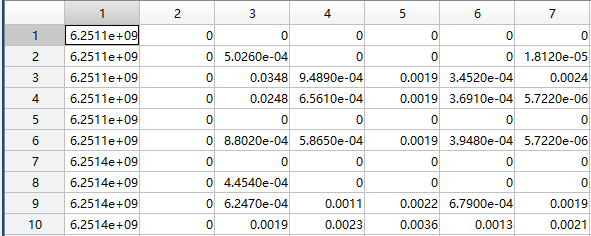inx数据格式
索引说明：对上述data的说明
每一个task的起始位置，以及序列长度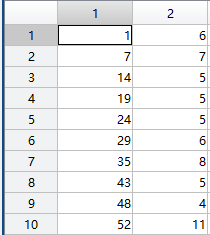源码
# -*- coding: utf-8 -*-
"""
用lstm 提取时间序列特征

input：一个task的序列 矩阵（jobid,taskid,5属性序列）
output：一个task时序特征 向量（jobid,taskid,时序特征）

time:2019-8-8
@author: bing
reference: https://blog.csdn.net/xiaokang06/article/details/80235950
"""

import tensorflow as tf
import numpy as np
import scipy.io as sio

#每次传入一个task矩阵
def get_tsfeatures(data):

jobid = data[0,0]      #jobid
taskid = data[0,1]     #taskid

dataA = data[:,2:7]    #5条序列
dataA = dataA.T
m,n = dataA.shape
dataA = dataA.reshape(m,1,n)          #转换成lstm的输入形式
dataA = tf.to_float(dataA)
X = dataA

X = tf.to_float(X)

def get_a_cell():
return tf.nn.rnn_cell.BasicLSTMCell(num_units=5)

#调用多层LSTM  range(1) 1个LSTM
stacked_lstm = tf.nn.rnn_cell.MultiRNNCell([get_a_cell() for _ in range(1)], state_is_tuple=True)

output, state = tf.nn.dynamic_rnn(stacked_lstm, X, time_major=False, dtype=tf.float32)

#设置占内存量
gpu_options = tf.GPUOptions(per_process_gpu_memory_fraction=0.333)
sess = tf.Session(config=tf.ConfigProto(gpu_options=gpu_options))
with tf.Session() as sess:

sess.run(tf.global_variables_initializer())
#        print('-------------------------state.h \n')    #将state.h作为时序特征
#        print(sess.run(state.h))

h = sess.run(state.h)       #tensor 转为 numpy
h1 = h.reshape(1,-1)           #变为1行n列  都是一个task的时序特征
#        print('-------------------------numpy:h1 \n')
#        print(h1)

tf.reset_default_graph()    #每次完成了，就清空tensor里的默认图、节点

sf = np.c_[jobid,taskid,h1]     #jobid,taskid,features   合并一起

return sf

if __name__ == "__main__":

sfeatures = []

data = sio.loadmat('C:/Users/bing/Desktop/LSTM/data.mat')['data']  #全部序列
inx = sio.loadmat('C:/Users/bing/Desktop/LSTM/numbs.mat')['numbs'] #task的索引，序列长度

for i in range(100):
if i==0:
data1 = data[inx[i,0]:(inx[i+1,0]-1)]     #按照索引 传入task矩阵【开始，结束】
sf = get_tsfeatures(data1)                 #获取时序特征
else:
data1 = data[inx[i,0]-1:(inx[i+1,0]-1)]   #[0:1],前闭后开，从第二个开始要判断一下
sf = get_tsfeatures(data1)

sfeatures.append(sf)                      #添加到列表里
print('{}/100 finished!'.format(i+1))

if i%10==0:    #100次 保存一下值
ss = np.array(sfeatures)
m,p,n = ss.shape
ss = np.array(sfeatures).reshape(m,n)
sio.savemat('h.mat',{"h":ss})


小白理解，不当之处望讨论
==================================================================
中间踩的坑
1、在服务器上跑程序时，内存爆炸的问题(TensorFlow的问题在于，默认情况下，它会在GPU启动时为其分配全部可用内存。)，解决办法：设置要分配内存数
    #设置占内存量
gpu_options = tf.GPUOptions(per_process_gpu_memory_fraction=0.333)
sess = tf.Session(config=tf.ConfigProto(gpu_options=gpu_options))

2、在用tensorflow 做循环的时候，每次循环完成后都需要清空图、节点，才能继续跑。（具体tf的知识了解较少，只是学过一点，过段时间研究生开学了再仔细学一遍）
tf.reset_default_graph()   #每次完成了，就清空tensor里的默认图、节点

3、昨晚跑的时候，计算了一下时间，提取一个task（一条序列）的时序特征大概需要20s,我共有170w条序列，嗯，跑完大概需要一年多，哈哈哈，忍不住自己都要笑了。
今天早上打开服务器看保存值时候，发现出了一点小bug,每次传入的是序列矩阵，但jobid,taskid并没有修改，修改后，速度提升了很快。本机上1s大概能提取2-3条序列的特征，服务器上会更好吧！
4、关于matlab 数据处理，两列的重复值问题，返回索引
原始：第一列索引
1 1 0 ···
2 1 0 ···
3 1 1 ···
4 1 2 ···
5 2 0 ···
6 2 0 ···
7 2 2 ···

我想要的：去掉重复行后的  索引值
1
3
4
5
7

一行代码，分别统计 两列 前后两行不为0的值。
inx = find([true;(diff(data(:,1))~=0) | (diff(data(:,2))~=0);true]);

5、保存数据的时候又除了问题，仔细看了一遍源码，并没有哪里出错，只是jobid,taskid的值不对，特征还是正确的，且按顺序的，解决办法是直接在matlab中选择出这些task
jt = data([numbs(:,1)]',[1,2]);   %取两列

6、数据精度失真的问题
jobid位数10+，用tf.float32时总显示不全，没法和其他数据样本进行匹配，后来换成float64好了，但是缺点是，内存容量增加了一倍


展开全文时间序列
• 2019-04-22 16:20:36 哈喽，大家好！我，人称神秘小马哥又回来了，不知道大家还记不记得上期我的...这期我给大家解密一下我三叉戟的第一根利器，LSTM模型，它在股价预测中更优于传统计量模型，并在语音识别，语...
https://www.toutiao.com/a6682630826011656711/

2019-04-22 16:20:36

哈喽，大家好！我，人称神秘小马哥又回来了，不知道大家还记不记得上期我的秘密三叉戟，轻松力压股市三大指数。这期我给大家解密一下我三叉戟的第一根利器，LSTM模型，它在股价预测中更优于传统计量模型，并在语音识别，语言翻译和智能营销等领域，也显示了其强大力量。1、解剖LSTM模型

LSTM模型是RNN模型的一个特殊例子，那RNN模型又是怎么一回事呢，别急，待我细细道来。RNN模型最主要的特性，即当前时间的状态不仅受到当前信息的影响，还受到之前状态的影响，我们先来看看下面这个结构：图表1 RNN结构

假设图中A为一段语音序列，X即表示这段语音中的一句话所含的信息，则第二句话不仅受自身所含信息X1的影响，还取决于第一句话所带的隐藏状态h0的影响。正因为此特性，RNN能够记忆序列本身信息，但因为RNN本身机制的设计，易导致严重的梯度爆炸和梯度消失问题（信息爆炸和后续信息丢失），从而记忆不了太长时间段信息，而且对内存和计算时间要求也高。鉴于此，LSTM提出了三扇“门”，遗忘门，输入门，输出门来解决RNN存在的问题。“遗忘门”——忘记部分过去的信息，“输入门”——记住部分现在的信息，然后将过去的记忆与现在的记忆合并后通过“输出门”——决定最终输出的部分。咦，人生不也如此嘛，忘记过去不开心的事情，记住当下美好的事物，才能生活的更美好（哎呀，一不小心，小马我撒了一下鸡汤，见谅）。下面我们言归正传，LSTM模型处理过程可如下图所示，Ct-1为上一阶段的信息状态，ht-1为上一阶段的隐藏状态，Xt为当前阶段所提供的信息。图表2遗忘门过程图表3输入门过程图表4细胞状态更新图表5输出门过程

以便大家理解，我再来给大家举个栗子，比如识别一段语音，X为其中一句话，我们在识别这句话时，会利用上一句话的信息帮助识别。假设上一句话的信息包含主题的性别，但此时这句话的信息中出现了新的性别，这时候“遗忘门”就起作用了，它会删去上句话中旧的主题性别，同时 “输入门”会更新新的主题性别。这样，当前信息状态即可得到一句新的输入。最终我们通过“输出门”决定输出哪部分信息，考虑到主题后可能出现的动词，它可能会输出主题的单复数信息，以便知道如何与动词结合在一起。通过对前期信息有选择的记忆和遗忘，LSTM实现了对相关信息的长期记忆，从而提取了时间特征。2、LSTM模型的用武之地

LSTM模型的神秘力量现在已经被运用于多个场景中，指数预测，语音识别，精准营销，哪哪儿都少不了它的身影，下面我给大家一一简单讲述一下。

2.1上证综指指数收益率预测

首先，先让我给你们讲讲我小马是如何用它的，以上证综指为例，我将其最高价，最低价，开盘价以及成交量和各类市值指标数据喂入模型，来预测其5日价格变动。此价格变动可为投资提供仓位和买卖时点的参考，具有很强的应用价值。我选取2016年1月1日到2018年9月13日的数据作为训练样本，2018年09与14日到2019年1月4日的数据作为测试样本。众所周知，A股市场在17年迎来了投资风格转变的一年，小盘股风光不再，而大盘股开始春风得意，如图6所示。图表6 指数走势

因此我们选择2016年到2019年3年数据，横跨两类投资风格，测试其对大盘指数收益率的预测效果，效果如下图。可见LSTM模型相较于传统线性模型ARIMAX对上证综指指数收益率的拟合轻松取得胜利。图表7 上证综指收益率预测

2.2语音识别

在语音识别方面，LSTM也是风头正劲。2018年谷歌老大哥发布了一新型全神经移动端 Gboard 语音识别器，其首先用于所有使用美式英语的 Pixel 手机。其中预测网络和编码器网络是 LSTM RNN，联合模型是前馈网络，这将谷歌语音的性能提升了50%。图表8 谷歌识别相同的语音句子时将服务器端语音识别器（左侧面板）与新型移动端识别器（右侧面板）进行对

2.3语言翻译图表9 谷歌GNMT翻译机架构

2016年，谷歌（又是它，崇拜脸）推出了其神经网络翻译系统（GNMT）。其在GNMT中，其编码层和解码层也都为LSTM结构。共有9层LSTM堆叠。其效果远好于当时最好水平的PBMT翻译系统，且在不同的任务翻译中，GNMT都已经接近人类专家水平。图表10 PNMT翻译准确率

2.4精准营销

UBT（User Behavior Tracking）即用户行为数据跟踪是LSTM模型的另一大主场。UBT即记录用户在APP上的各类行为，包括设备、网络情况，软件环、地理位置分布、操作习惯等，数据具有非常大的挖掘价值，但同时挖掘难度也很大。相比于传统的建模方式(数据准备 -> 特征提取 -> 模型训练)，LSTM模型不仅能抓住某些特定的操作模式，还能记住用户历史的操作行为，在UBT这种和时间序列相关的案例中LSTM模型完胜。

以某信贷产品精准营销为例，LSTM模型以该产品现有借款用户在APP上的海量数据来训练，根据其是否逾期的表现，得到对用户风险评估有较好区分度的风险模型。该模型对此产品申请借款用户实现了很好的风险区分度。图表11 某信贷产品LSTM精准营销框架

听完我的碎碎念，相信大家现在对LSTM模型有了一个大概的了解了吧。下期我会给大家讲我三叉戟的第二根利器，CNN模型，敬请期待！关注兴业数金微信公众号，了解更多金融信息服务。

展开全文• CNN与LSTM分别作为两个通道，通过注意力机制（attention）融合，从而使模型能同时表达数据在空间维度和时间维度上的特征，并以时间序列预测的方式验证融合模型特征提取的有效性。实验结果表明，双通道融合模型与单一...
•  outputs, final_state = tf.nn.dynamic_rnn(lstm_cell, X_in, initial_state=init_state, time_major=False)  results = tf.matmul(final_state, weights['out']) + biases['out'] # 2 ndim  dim = results....
# -*- coding: utf-8 -*-
# read the data of npy,the data is input(label)
import os
import numpy as np
import tensorflow as tf
import time
import math

batch_size = 128
path =os.getcwd()
keep_prob = tf.placeholder(tf.float32)

# In order to get the suitable type of data and label
def initial_data(all_data,all_label): #data and label are all the name of .npy
Data_Examples = np.load(path+'/'+ all_data)   # all_data = .npy
Data_Labels = np.load(path+'/'+ all_label)
Data_Labels = tf.one_hot(Data_Labels,2,1,0)
Data_Labels = tf.to_float(Data_Labels,name = 'Data_Labels')
return Data_Examples,Data_Labels

def get_batch_data(t_enh,t_enh_label,t_pro,t_pro_label):
t_enh = tf.cast(t_enh, tf.float32)
t_enh_label = tf.cast(t_enh_label, tf.float32)
t_pro = tf.cast(t_pro, tf.float32)
t_pro_label = tf.cast(t_pro_label, tf.float32)

input_queue = tf.train.slice_input_producer([t_enh,t_enh_label,t_pro,t_pro_label], shuffle=False)
t_enh_b,t_enh_label_b,t_pro_b,t_pro_label_b = tf.train.batch(input_queue, batch_size=batch_size, num_threads=2,                                                                                       capacity=20000)
return t_enh_b,t_enh_label_b,t_pro_b,t_pro_label_b

# train_batch_holder#
train_enh_batch_holder = tf.placeholder(tf.float32,shape=(None,10001,4,1),name = 'x-input')
train_pro_batch_holder = tf.placeholder(tf.float32,shape=(None,10001,4,1),name = 'x2-input')
train_enh_label_holder = tf.placeholder(tf.float32,shape=(None,2),name = 'y-input')

def cnn_1(train_batch_holder,filter_size_1,filter_number_1,pool_1_size):
filters_1 = tf.Variable(tf.random_normal([filter_size_1,4,1,filter_number_1],dtype=tf.float32,seed=1))
biases_1 = tf.Variable(tf.random_normal([filter_number_1],dtype=tf.float32,seed=1))
conv_1 =tf.nn.conv2d(train_batch_holder,filters_1,strides=[1,1,1,1],padding='VALID')    #####train_batch_holder#####
bias_1 = tf.nn.bias_add(conv_1,biases_1)
actived_conv_1 = tf.nn.relu(bias_1)
pool_1 = tf.nn.max_pool(actived_conv_1,ksize=[1,pool_1_size,1,1],strides=[1,pool_1_size,1,1],padding="VALID")
return pool_1

def cnn_2(pool_1,filter_size_2,filter_number_1,filter_number_2,pool_2_size):
filters_2 = tf.Variable(tf.random_normal([filter_size_2,1,filter_number_1,filter_number_2],seed=1))
biases_2 = tf.Variable(tf.random_normal([filter_number_2],dtype=tf.float32))
conv_2 = tf.nn.conv2d(pool_1,filters_2,strides=[1,1,1,1],padding='VALID')           # input pool_1
bias_2 = tf.nn.bias_add(conv_2,biases_2)
actived_conv_2 = tf.nn.relu(bias_2)
pool_2 = tf.nn.max_pool(actived_conv_2,ksize=[1,pool_2_size,1,1],strides=[1,pool_2_size,1,1],padding="VALID")
return pool_2

def cat_cnn(pool_2_enh,pool_2_pro):    # cat the enh_pro for next rnn
pool_cat = tf.concat([pool_2_enh,pool_2_pro],1)
pool_cat_1_ndim = pool_cat.get_shape().value
pool_cat_2_ndim = pool_cat.get_shape().value
pool_cat_4_ndim = pool_cat.get_shape().value
pool_cat_3ndim = tf.reshape(pool_cat,[-1,pool_cat_2_ndim,pool_cat_4_ndim])
x = pool_cat_3ndim
return x,pool_cat_2_ndim,pool_cat_4_ndim

def rnn_1(x, pool_cat_2_ndim,pool_cat_4_ndim,filter_size_1,filter_size_2):
n_input = pool_cat_2_ndim  # w
n_step = pool_cat_4_ndim # h
n_hidden = 128
n_classes = 2
weights = {'in': tf.Variable(tf.random_normal([n_input, n_hidden])),
'out': tf.Variable(tf.random_normal([n_hidden, n_classes]))}
biases = {'in': tf.Variable(tf.constant(0.1, shape=[n_hidden])),
'out': tf.Variable(tf.constant(0.1, shape=[n_classes]))}
X = tf.reshape(x, [-1, n_input])
X_in = tf.matmul(X, weights['in'])+biases['in']
X_in = tf.reshape(X_in, [-1, n_step, n_hidden])
#define LSTMcell
lstm_cell = tf.contrib.rnn.BasicLSTMCell(n_hidden)
with tf.variable_scope("lstm_cell"+str(filter_size_1)+'_'+str(filter_size_2), reuse=None):
init_state = lstm_cell.zero_state(batch_size, dtype=tf.float32)
outputs, final_state = tf.nn.dynamic_rnn(lstm_cell, X_in, initial_state=init_state, time_major=False)
results = tf.matmul(final_state, weights['out']) + biases['out']    # 2 ndim
dim = results.get_shape().value
return results,dim

#以上是对.npy数据的读入操作，以及接入CNN，RNN等操作，后续部分接入全连接层与计算 ACC等是常规流程，在此不做赘述。
展开全文tensorflow
• 基于双通道LSTM的步态多特征提取用于诊断神经退行性疾病研究论文
• 同时阅读了关于多模态以及特征融合的论文，使用LSTM-Attention和CNN进行文档尺寸特征提取，同时结合Mairesse语言学特征，将多个分割提取的特征融合起来进行分析，最终使用Pytorch进行模型实现。 其中4个类别的提升...Python
• 代码详解 代码总共包括两个.m文件 read_data_1800_High.m main_1800_High.m 其中第一个用于读取并划分原始数据 第二个用于完成划分训练集测试集，特征提取+分类等工作 2.1 read_data_1800_High // 本函数Input为 //...
1. 数据描述
齿轮箱数据来自PHM2009年的数据挑战赛，官网：PHM2009数据挑战赛。所测试的齿轮包括一组直齿轮和斜齿轮，本例中用直齿轮的数据进行验证。实验设备照片如下。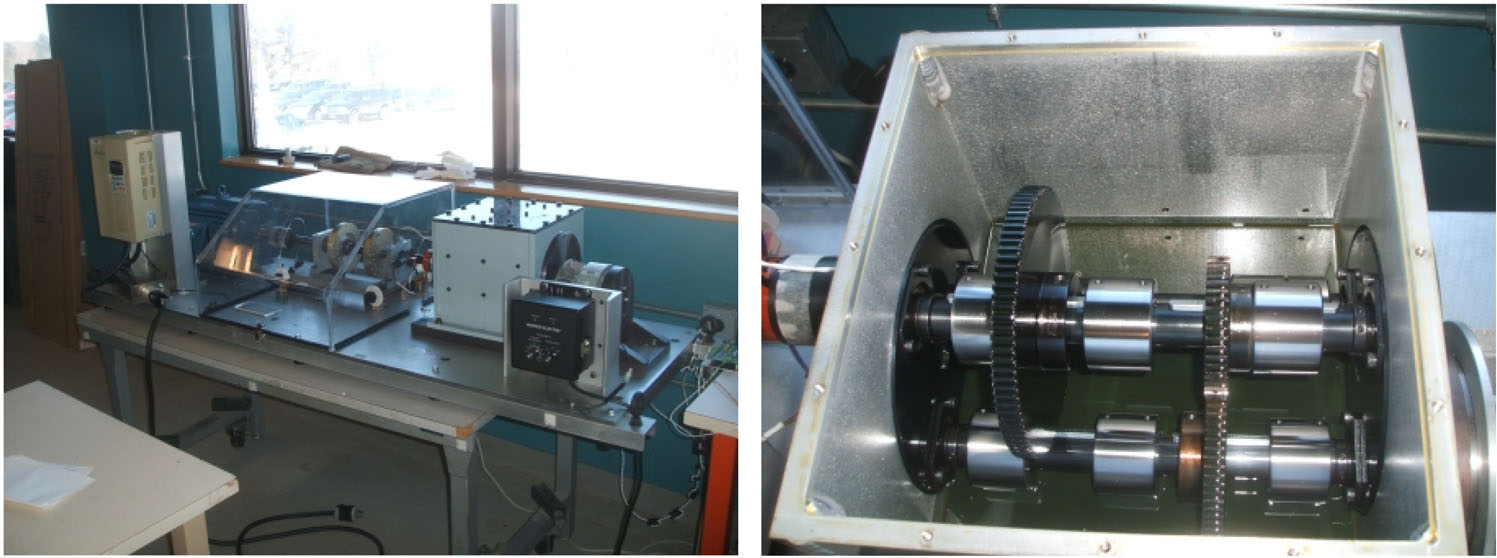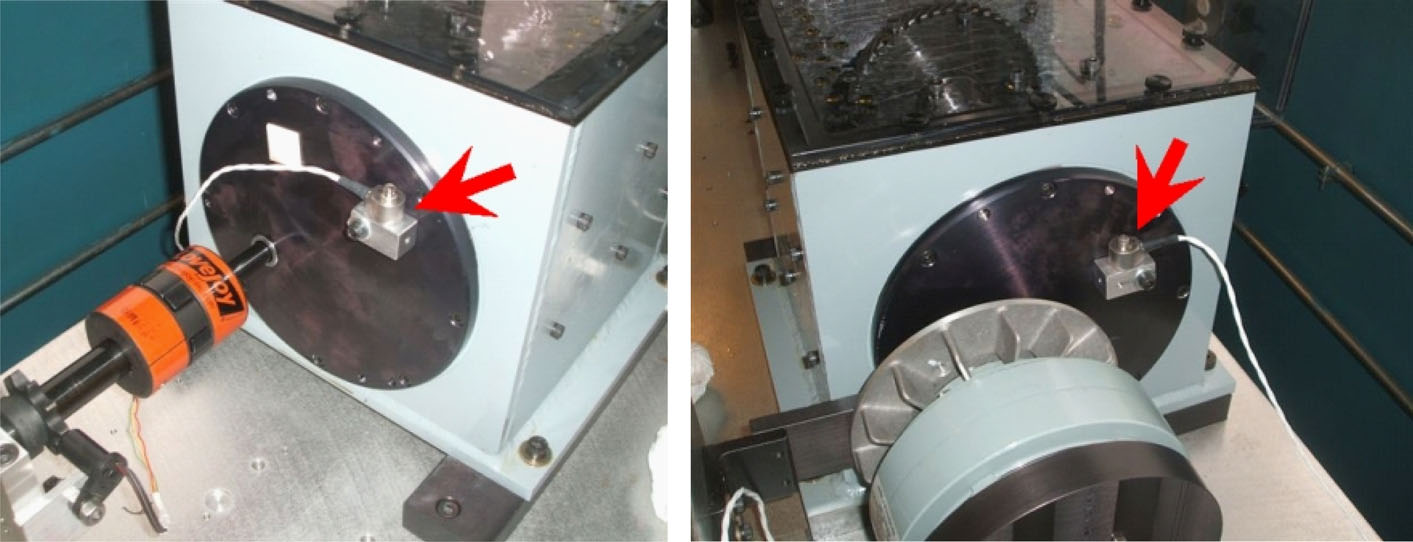齿轮箱的输入侧和输出侧各安装一个加速度传感器，传感器参数：灵敏度：10mv/g，采样速率66.67KHz。所用的采集卡采集三个通道的数据，分别为:

通道1：输入侧振动传感器数据
通道2：输出侧振动传感器数据
通道3：转速信号

采用人工注入故障的方式，共有8种故障类型，如下表。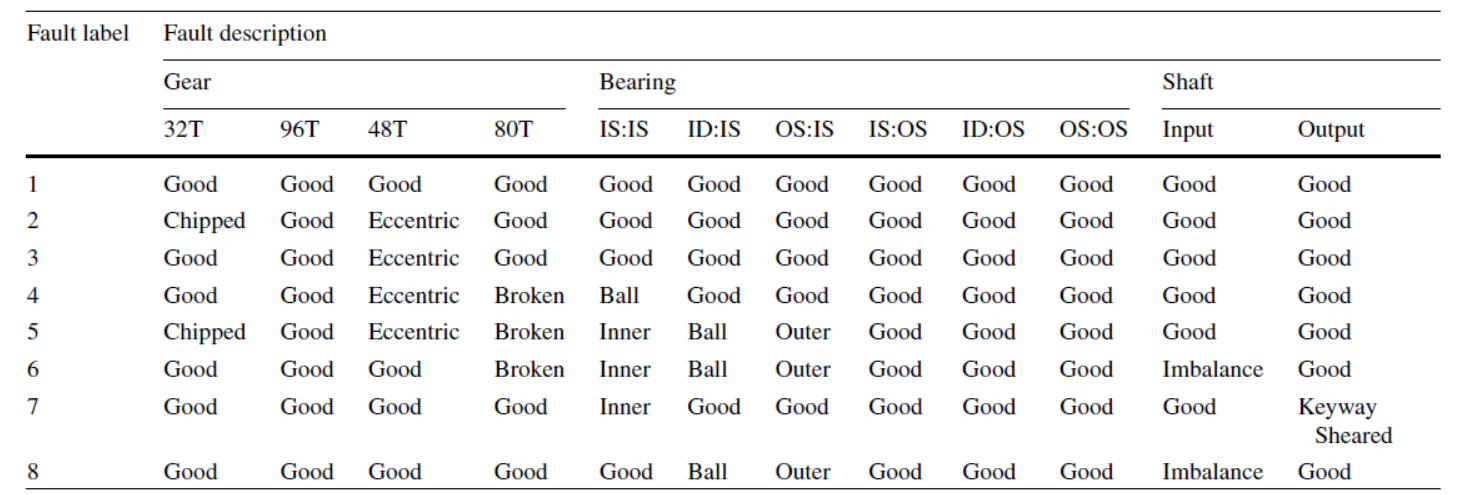实验时工况为：转速分别设置为1800rpm, 2100rpm, 2400rpm, 2700rpm, 3000rpm，负载分别为低载和高载，交叉后共有10种工况，分别为
1800 rpm | 低载，1800rpm|高载，以此类推。
本例采用1800rpm|高载的实验数据建模及验证，采用通道二即输出侧振动传感器数据。每个工况下每种故障类型数据采集持续8秒，因此对于每种故障类型，每个通道总共得到66.7KHz×8秒=533307个数据点。每种故障类型的数据为533307×3通道的矩阵，数据在matlab打开如下。把通道2的数据plot出来，如下所示。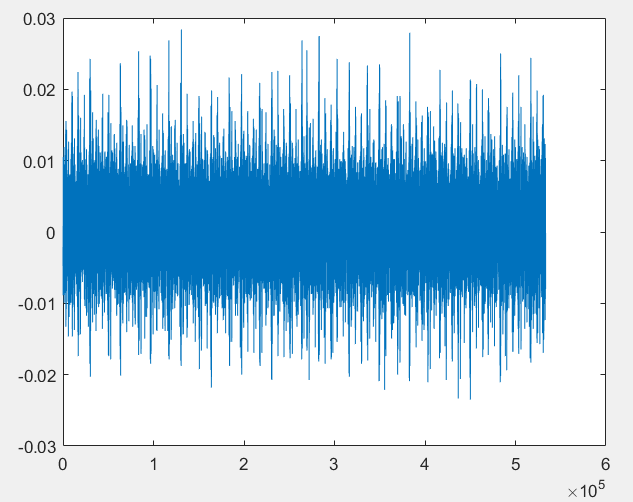2. 代码详解
代码总共包括两个.m文件

read_data_1800_High.m
main_1800_High.m
其中第一个用于读取并划分原始数据
第二个用于完成划分训练集测试集，特征提取+分类等工作

2.1 read_data_1800_High
// 本函数Input为
// interval - 数据划分长度，默认为6400，即每6400个数据点划为一个样本
// ind_column - 通道，默认为2，即选取第二个通道
// Output为
// label1 label2, ..., label8，分别为划分好的8种故障类型的样本
%
function [label1 label2 label3 label4 label5 label6 label7 label8]= read_data_1800_High( interval, ind_column )
if nargin <2
ind_column=2; //如果传递的实参小于2个，默认ind_column为2
end

if nargin <1
interval=6400; //默认interval=6400
end

file_rul='E:\Datasets\PHM data challenge\2009 PHM Society Conference Data Challenge-gear box\spur_30hz_High\';
// 以下为获取file_rul路径下.mat格式的所有文件
file_folder=fullfile(file_rul);
dir_output=dir(fullfile(file_folder,'*.mat'));
file_name={dir_output.name}';
num_file=max(size(file_name)); //num_file为文件数，本例中num_file=8，8个文件，分别存储齿轮箱的8种故障数据

for i=1:num_file
file=[file_rul,file_name{i}];
load(file);
[filepath, name, ext]=fileparts(file);
raw=eval(name);

// 每6400个点划分为一个样本
n=1;
left_index=1+(n-1)*interval;
right_index=n*interval;
while right_index<=size(raw,1)
temp=raw(left_index : right_index, ind_column);
// eval函数构造label1, label2,...等变量名
eval(['label' num2str(i) '(:,n)=temp;']);
n=n+1;
left_index=1+(n-1)*interval;
right_index=n*interval;
end
end
end

2.2 main_1800_High
// 读取数据，label1为一个6400*83的数组，83为每种故障类型所得到的样本数
[label1, label2, label3, label4, label5, label6, label7, label8]=read_data_1800_High();
num_categories=8;
// 由于matlab中LSTM建模需要，用num2cell函数将label1转为cell型，label_x_cell为一个1×83的cell型数组，每个cell存储6400个数据点
label1_x_cell=num2cell(label1,1);
label2_x_cell=num2cell(label2,1);
label3_x_cell=num2cell(label3,1);
label4_x_cell=num2cell(label4,1);
label5_x_cell=num2cell(label5,1);
label6_x_cell=num2cell(label6,1);
label7_x_cell=num2cell(label7,1);
label8_x_cell=num2cell(label8,1);

num_1=length(label1_x_cell);
num_2=length(label2_x_cell);
num_3=length(label3_x_cell);
num_4=length(label4_x_cell);
num_5=length(label5_x_cell);
num_6=length(label6_x_cell);
num_7=length(label7_x_cell);
num_8=length(label8_x_cell);
// 创建用于存储每种故障类型的标签的数据结构，由于matlab中lstm建模需要，也需要cell型数据。例如，label1_y为一个83×1的cell型数组，目前其值为空
label1_y=cell(num_1,1);
label2_y=cell(num_2,1);
label3_y=cell(num_3,1);
label4_y=cell(num_4,1);
label5_y=cell(num_5,1);
label6_y=cell(num_6,1);
label7_y=cell(num_7,1);
label8_y=cell(num_8,1);
// 创建故障类型的标签，用1,2,3,...,8表示8种故障标签，给对应标签赋值。
for i=1:num_1; label1_y{i}='1'; end
for i=1:num_2; label2_y{i}='2'; end
for i=1:num_3; label3_y{i}='3'; end
for i=1:num_4; label4_y{i}='4'; end
for i=1:num_5; label5_y{i}='5'; end
for i=1:num_6; label6_y{i}='6'; end
for i=1:num_7; label7_y{i}='7'; end
for i=1:num_8; label8_y{i}='8'; end
// 用dividerand函数将每种故障类型的数据随机划分为4:1的比例，分别用作训练和测试
[trainInd_label1,~,testInd_label1]=dividerand(num_1,0.8,0,0.2);
[trainInd_label2,~,testInd_label2]=dividerand(num_2,0.8,0,0.2);
[trainInd_label3,~,testInd_label3]=dividerand(num_3,0.8,0,0.2);
[trainInd_label4,~,testInd_label4]=dividerand(num_4,0.8,0,0.2);
[trainInd_label5,~,testInd_label5]=dividerand(num_5,0.8,0,0.2);
[trainInd_label6,~,testInd_label6]=dividerand(num_6,0.8,0,0.2);
[trainInd_label7,~,testInd_label7]=dividerand(num_7,0.8,0,0.2);
[trainInd_label8,~,testInd_label8]=dividerand(num_8,0.8,0,0.2);

// 构建每种故障类型的训练数据
xTrain_label1=label1_x_cell(trainInd_label1);
yTrain_label1=label1_y(trainInd_label1);

xTrain_label2=label2_x_cell(trainInd_label2);
yTrain_label2=label2_y(trainInd_label2);

xTrain_label3=label3_x_cell(trainInd_label3);
yTrain_label3=label3_y(trainInd_label3);

xTrain_label4=label4_x_cell(trainInd_label4);
yTrain_label4=label4_y(trainInd_label4);

xTrain_label5=label5_x_cell(trainInd_label5);
yTrain_label5=label5_y(trainInd_label5);

xTrain_label6=label6_x_cell(trainInd_label6);
yTrain_label6=label6_y(trainInd_label6);

xTrain_label7=label7_x_cell(trainInd_label7);
yTrain_label7=label7_y(trainInd_label7);

xTrain_label8=label8_x_cell(trainInd_label8);
yTrain_label8=label8_y(trainInd_label8);

// 构建每种故障类型的测试数据
xTest_label1=label1_x_cell(testInd_label1);
yTest_label1=label1_y(testInd_label1);

xTest_label2=label2_x_cell(testInd_label2);
yTest_label2=label2_y(testInd_label2);

xTest_label3=label3_x_cell(testInd_label3);
yTest_label3=label3_y(testInd_label3);

xTest_label4=label4_x_cell(testInd_label4);
yTest_label4=label4_y(testInd_label4);

xTest_label5=label5_x_cell(testInd_label5);
yTest_label5=label5_y(testInd_label5);

xTest_label6=label6_x_cell(testInd_label6);
yTest_label6=label6_y(testInd_label6);

xTest_label7=label7_x_cell(testInd_label7);
yTest_label7=label7_y(testInd_label7);

xTest_label8=label8_x_cell(testInd_label8);
yTest_label8=label8_y(testInd_label8);

// 将每种故障类型的数据整合，构建完整的训练集和测试集
xTrain=[xTrain_label1 xTrain_label2 xTrain_label3 xTrain_label4 xTrain_label5 xTrain_label6 xTrain_label7 xTrain_label8];
yTrain=[yTrain_label1; yTrain_label2; yTrain_label3; yTrain_label4; yTrain_label5; yTrain_label6; yTrain_label7; yTrain_label8];
num_train=size(xTrain,2);

xTest=[xTest_label1  xTest_label2  xTest_label3 xTest_label4 xTest_label5 xTest_label6 xTest_label7 xTest_label8];
yTest=[yTest_label1; yTest_label2; yTest_label3; yTest_label4; yTest_label5; yTest_label6; yTest_label7; yTest_label8];
num_test=size(xTest,2);

//================================================================================
//以下分别对每个样本，提取三种特征：1.瞬时频率，2.瞬时谱熵，3.小波包能量，
//上述三种特征后面会被送入分类器中进行分类，实验结果表明，将小波包能量作为特征，
//能够取得最高的分类精度

// 提取瞬时频率：用matlab的pspectrum对每个样本进行谱分解，再用instfreq函数计算瞬时频率
FreqResolu=25;
TimeResolu=0.12;
// the output of pspectrum 'p' contains an estimate of the short-term, time-localized power spectrum of x.
// In this case, p is of size Nf × Nt, where Nf is the length of f and Nt is the length of t.
[p,f,t]=cellfun(@(x) pspectrum(x,fs,'TimeResolution',TimeResolu,'spectrogram'),xTrain,'UniformOutput', false);
instfreqTrain=cellfun(@(x,y,z) instfreq(x,y,z)', p,f,t,'UniformOutput',false);
[p,f,t]=cellfun(@(x) pspectrum(x,fs,'TimeResolution',TimeResolu,'spectrogram'),xTest,'UniformOutput', false);
instfreqTest=cellfun(@(x,y,z) instfreq(x,y,z)', p,f,t,'UniformOutput',false);

// 提取瞬时谱熵：用matlab的pspectrum对每个样本进行谱分解，再用pentropy函数计算瞬时频率
[p,f,t]=cellfun(@(x) pspectrum(x,fs,'TimeResolution',TimeResolu,'spectrogram'),xTrain,'UniformOutput', false);
pentropyTrain=cellfun(@(x,y,z) pentropy(x,y,z)', p,f,t,'UniformOutput',false);
[p,f,t]=cellfun(@(x) pspectrum(x,fs,'TimeResolution',TimeResolu,'spectrogram'),xTest,'UniformOutput', false);
pentropyTest=cellfun(@(x,y,z) pentropy(x,y,z)', p,f,t,'UniformOutput',false);

// 提取小波包能量
// num_level=5表示进行小波包五层分解，共获得2^5=32个值组成的特征向量。
num_level=5;
index=0:1:2^num_level-1;
// wpdec为小波包分解函数
treeTrain=cellfun(@(x) wpdec(x,num_level,'dmey'), xTrain, 'UniformOutput', false);
treeTest=cellfun(@(x) wpdec(x,num_level,'dmey'), xTest, 'UniformOutput', false);
for i=1:num_train
for j=1:length(index)
// wprcoef为小波系数重构函数
reconstr_coef=wprcoef(treeTrain{i},[num_level,index(j)]);
// 计算能量
energy(j)=sum(reconstr_coef.^2);
end
energyTrain_doule(i,:)=energy;
end

energyTrain=num2cell(energyTrain_doule,2);
energyTrain=energyTrain';

for i=1:num_test
for j=1:length(index)
reconstr_coef=wprcoef(treeTest{i},[num_level,index(j)]);
energy(j)=sum(reconstr_coef.^2);
end
energyTest_double(i,:)=energy;
end

energyTest=num2cell(energyTest_double,2);
energyTest=energyTest';

// ===============组装用于送入分类器的特征序列====================
// 下面的语句仅用了小波包能量作为输入特征
xTrainFeature=cellfun(@(x)[x], energyTrain', 'UniformOutput',false);
xTestFeature=cellfun(@(x)[x], energyTest', 'UniformOutput',false);
// 如果想用 瞬时谱熵和小波包能量三种特征作为输入，如下
// xTrainFeature=cellfun(@(x,y,z)[x;y;z],energyTrain',instfreqTrain', pentropyTrain', 'UniformOutput',false);
// xTestFeature=cellfun(@(x,y,z)[x;y;z], energyTest',instfreqTest',pentropyTest', 'UniformOutput',false);

// ============================数据标准化================================
XV=[xTrainFeature{:}];
mu=mean(XV,2);
sg=std(XV,[],2);

xTrainFeatureSD=xTrainFeature;
xTrainFeatureSD=cellfun(@(x)(x-mu)./sg, xTrainFeatureSD,'UniformOutput',false);

xTestFeatureSD=xTestFeature;
xTestFeatureSD=cellfun(@(x)(x-mu)./sg,xTestFeatureSD,'UniformOutput',false);

// =========================设计LSTM网络=================================
yTrain_categorical=categorical(yTrain);
numClasses=numel(categories(yTrain_categorical));
yTest_categorical=categorical(yTest);
sequenceInput=size(xTrainFeatureSD{1},1); // 如果选了3种特征作为数据，这里改为"3"

// 创建用于sequence-to-label分类的LSTM步骤如下：
// 1. 创建sequence input layer
// 2. 创建若干个LSTM layer
// 3. 创建一个fully connected layer
// 4. 创建一个softmax layer
// 5. 创建一个classification outputlayer
// 注意将sequence input layer的size设置为所包含的特征类别数，本例中，1或2或3，取决于你用了几种特征。fully connected layer的参数为分类数，本例中为8.
layers = [ ...
sequenceInputLayer(sequenceInput)
lstmLayer(256,'OutputMode','last')
fullyConnectedLayer(numClasses)
softmaxLayer
classificationLayer
];

maxEpochs=600;
miniBatchSize=32;
// 如果不想展示训练过程，
options = trainingOptions('adam', ...
'ExecutionEnvironment', 'gpu',...
'SequenceLength', 'longest',...
'MaxEpochs',maxEpochs, ...
'MiniBatchSize', miniBatchSize, ...
'InitialLearnRate', 0.001, ...
'GradientThreshold', 1, ...
'plots','training-progress', ...
'Verbose',true);

// ======================训练网络=========================
net2 = trainNetwork(xTrainFeatureSD,yTrain_categorical,layers,options);
// ======================测试网路==========================
testPred2 = classify(net2,xTestFeatureSD);
// 打印混淆矩阵
plotconfusion(yTest_categorical',testPred2','Testing Accuracy')

训练过程截图，如果不想展示该图，将options中的 'plots','training-progress', ... 改为'plots','none', ...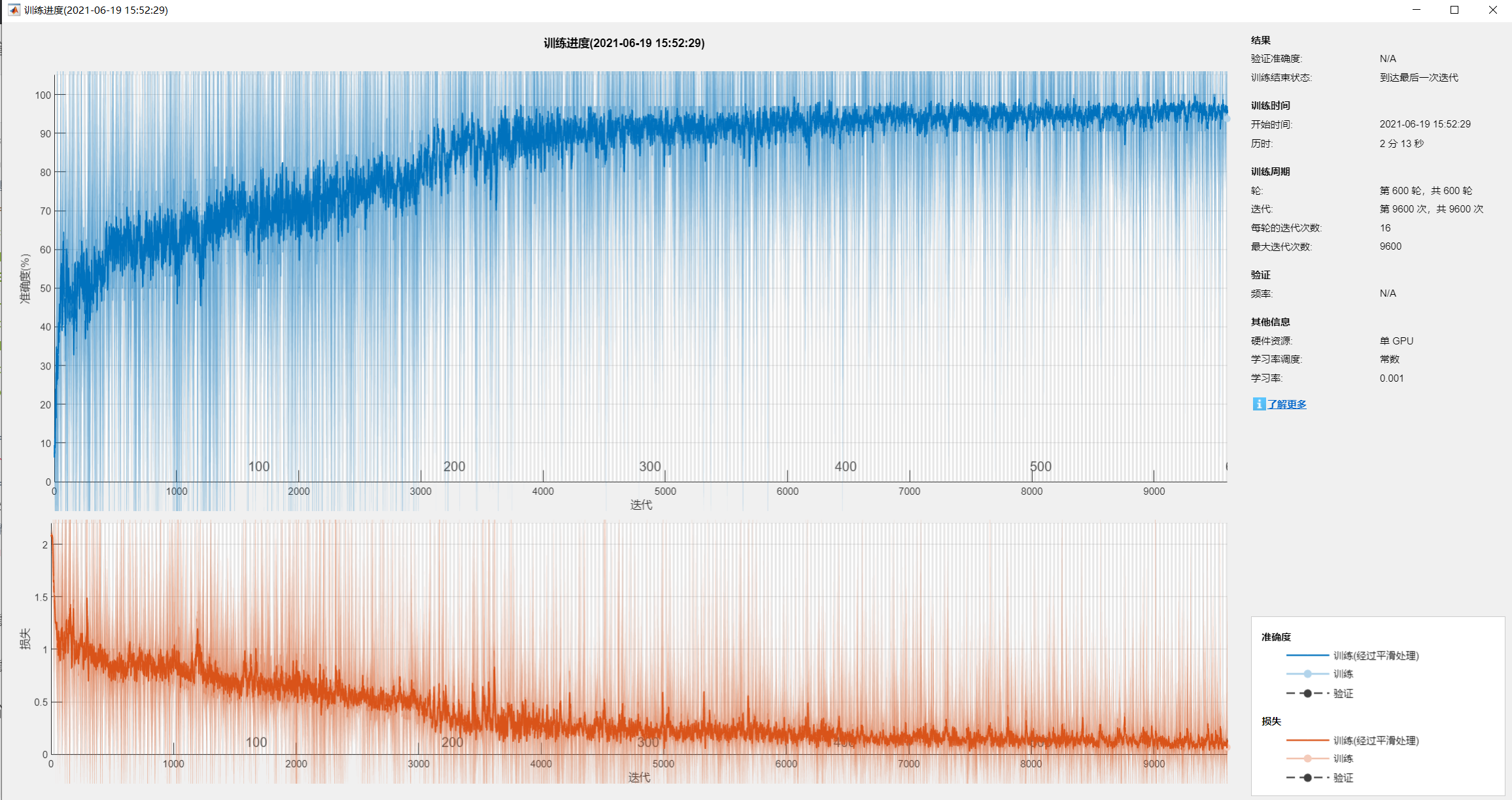混淆矩阵截图，可以看到，精度为94%。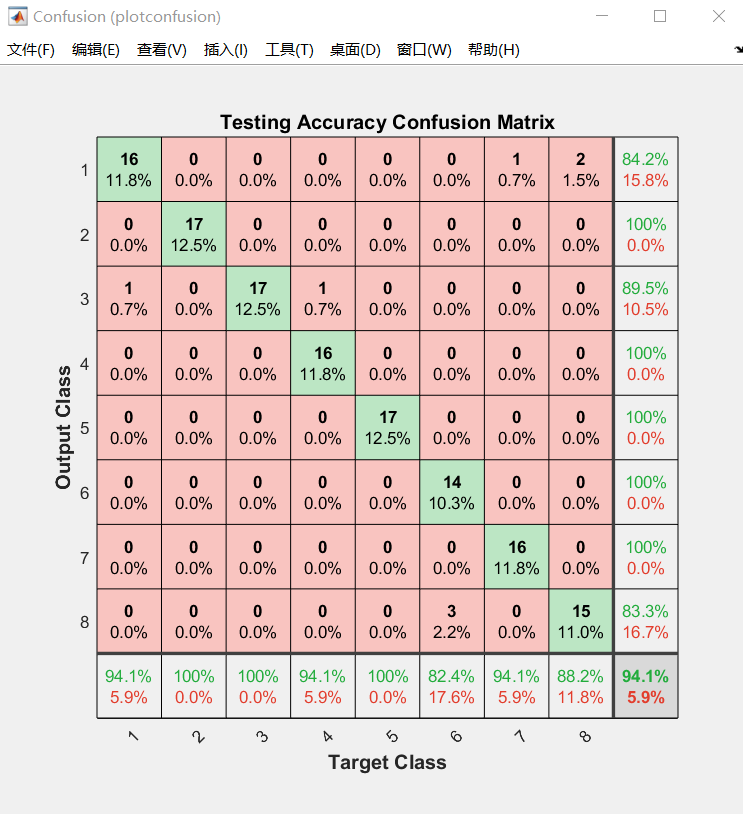将上述两个文件放在同一个workspace下，运行main_1800_High即可
3 展望
在后续的研究中，我们发现在keras深度学习框架下用CNN做此类问题更简便，不需要进行特征提取，教程后续补上。


展开全文• 2.经由BILSTM特征提取，输出是每个单词对应的预测标签。 3.经CRF层约束，会对输出的分数进行校正，输出是最优标签序列。 Step1：Word Embedding 定义：Word Embedding是一个基于分布式假设的预训练模型。它假设两个...自然语言处理 神经网络 深度学习 机器学习 人工智能
• 它使用两个CNN之一来提取图像特征，然后将其输入LSTM。 然后，LSTM逐字生成一个句子。 该项目基于使用TensorFlow 1.14的Python 3.7.4构建。 Inception_v3和VGG16是此项目中使用的两个预加密的CNN。 它使用Flickr30k...
• 包含数据清洗，数据特征提取，数据建模，数据预测。 想学习更多深度学习项目，可访问如下链接 1.通过自回归(AR,ARIMA)模型进行时间序列预测合集：https://github.com/yangwohenmai/TimeSeriesForecasting 2.通过...
• 提取均值信号特征的matlab代码两个扬声器的基于 LSTM/BLSTM 的 PIT 在多通话者混合语音分离和识别方面取得的进展，通常被称为“鸡尾酒会问题”，并没有那么令人印象深刻。 尽管人类听众可以很容易地感知混合声音中的...
• 目录 一、简介 二、T-LSTM ...F-LSTM和TF-LSTM主要目的是为了取代CNN作为特征提取的工具，来达到对频带间相关性建模的目的。F-LSTM和TF-LSTM也是通过类似卷积的方式，通过一个小的滑动窗来捕获语音...
• ## 利用python实现语音文件的特征提取

万次阅读 多人点赞 2018-07-06 15:33:17
概述 语音识别是当前人工智能的比较热门的方向，...但训练这些模型的第一步就是将音频文件数据化，提取当中的语音特征。 MP3文件转化为WAV文件 录制音频文件的软件大多数都是以mp3格式输出的，但mp3格式文...语音识别 Python
• ## LSTM

2019-09-25 02:49:08
循环神经网络在网络中引入了... RNN引入了隐状态h(hidden state)的概念,h可以对序列形的数据提取特征，接着再转换为输出。每步的参数都是共享的 在经典的RNN结构中，通常使用tanh作为激活函数。 存在以下结构： ...
• 图像字幕生成器 一个Web应用程序，用于为图像生成标题。 VGG-16模型用于对图像进行编码和提取特征，而LSTM将用于训练字幕。
• 但训练这些模型的第一步就是将音频文件数据化，提取当中的语音特征。 MP3文件转化为WAV文件 录制音频文件的软件大多数都是以mp3格式输出的，但mp3格式文件对语音的压缩比例较重，因此首先利用ffmpeg将转化为wav原始...
• 另外一种方法就是来从时间序列中提取特征从而将这些特征用在有监督的模型上。在这篇文章中，我们来看下如何使用tsfresh包自动的提取时间序列数据。 这份数据的来源是 。这个网站中提供对很多数据集有高精度的模型，...时间序列 数据提取
• 最近在复现ubuntu dialogue corpus 中用到的一些语义匹配模型的方法。 先是试了试siamese lstm network。...为了提取出context，和response的语义信息，模型采用了共享参数的lstm提取特征。然后用了双线性的方法...
• 特征提取 深度学习方法例子 5.1 LSTM 5.2 GRU 5.3 CNN 5.4 GIN 第3篇 特征提取 特征提取是指通过专业知识从输入信号中提取区别特征的过程。 从时域（例如，方差，平均值，峰度），频域（例如，快速傅立叶变换）和时...深度学习 机器学习 大数据 算法 人工智能
• 图像字幕生成器：LSTM模型从预先训练的VGG-16模型中提取特征后，会为输入图像生成字幕。 （计算机视觉，自然语言处理，深度学习，Python）
• 项目和资料汇总参考项目汇总kaggle项目天池项目深度学习学习资料汇总机器学习总流程sklearn细节问题信号处理信号特征及特征提取方法 参考项目汇总 kaggle项目 【VSB 电源线故障检测】CNN + LSTM用于信号分类： ...
• 现有方法利用模式，约束和机器学习技术来提取因果关系，这在很大程度上取决于领域知识，并且需要相当多的人力和时间来进行特征工程。 在本文中，我们基于新的因果关系标记方案，将因果关系提取公式指定为序列标记...
• ## lstm进行多变量预测

千次阅读 2020-06-09 21:25:48
「公开课」LSTM长短期记忆神经网络的学习与实现 什么是LSTM? 长短期记忆（Long short-term memory, LSTM）是一种特殊的RNN，...用LSTM提取时序特征的优越性 VS传统的特征提取：HOG， LBP ...
• 用于中文命名实体识别的递归神经网络 命名实体识别作为序列...汉字序列被投影到密集的向量序列中，并带有多余的特征作为循环层的输入，这里我们使用一个代表词边界特征的热向量进行说明。循环层是双向LSTM层，前向和pytorch 自然语言处理 神经网络
• 2019年上半年收集到的人工智能LSTM干货文章 门控神经网络：LSTM 和 GRU 简要...LSTM-CNN-Attention算法系列之一：LSTM提取时间特征 对时间序列分类的LSTM全卷积网络的见解 2019-06-24 写于苏州市。 ...
• 然后研究了在特征空间中对说话人进行建模的技术，提取出能有效反映说话人和信道信息的身份认证矢量（i-vector）；最后将上述研究结合构建了基于i-vector特征LSTM递归神经网络系统。在Open KWS 2013标准数据集上...语音识别 长短时记忆神经网络...